UIHistories Project: A History of the University of Illinois by Kalev Leetaru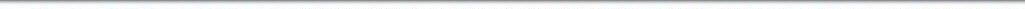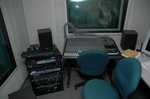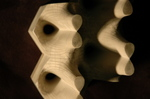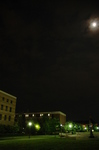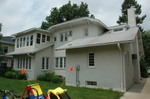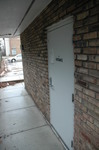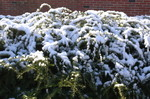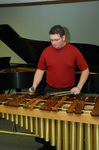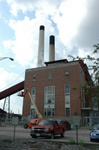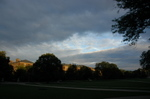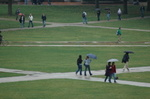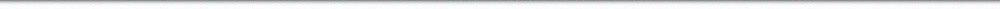N A V I G A T I O N D I G I T A L L I B R A R Y

## Repository: UIHistories Project: Board of Trustees Minutes - 1896 [PAGE 209]

Caption: Board of Trustees Minutes - 1896
This is a reduced-resolution page image for fast online browsing.

< Previous Page [Displaying Page 209 of 371] Next Page >
[VIEW ALL PAGE THUMBNAILS]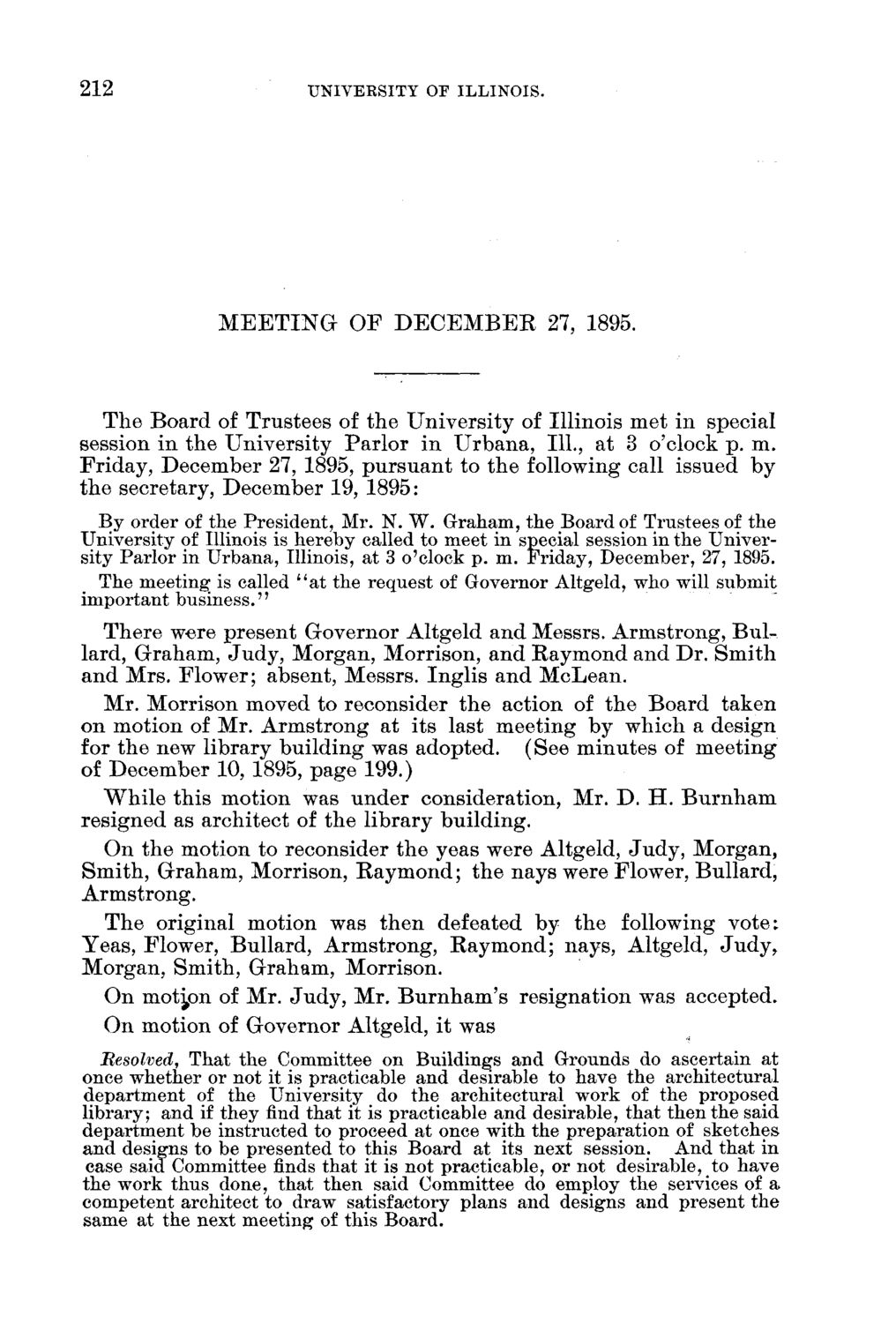### EXTRACTED TEXT FROM PAGE:

212

UNIVERSITY OF ILLINOIS.

M E E T I N G O F D E C E M B E R 27, 1895.

T h e B o a r d of T r u s t e e s of t h e U n i v e r s i t y of I l l i n o i s m e t in special session in t h e U n i v e r s i t y P a r l o r i n U r b a n a , 111., at 3 o'clock p . m . F r i d a y , D e c e m b e r 27, 1895, p u r s u a n t to t h e following call issued b y t h e secretary, D e c e m b e r 19, 1895: By order of the President, Mr. N. W. Graham, the Board of Trustees of the University of Illinois is hereby called to meet in special session in the University Parlor in Urbana, Illinois, at 3 o'clock p. m. Friday, December, 27, 1895. The meeting: is called " a t the request of Governor Altgeld, who will submit important business. 77 T h e r e were p r e s e n t G o v e r n o r A l t g e l d a n d Messrs. A r m s t r o n g , B u l lard, G r a h a m , J u d y , M o r g a n , M o r r i s o n , a n d R a y m o n d a n d D r . S m i t h a n d M r s . F l o w e r ; absent, Messrs. I n g l i s a n d M c L e a n . Mr. M o r r i s o n moved to reconsider t h e action of t h e B o a r d t a k e n o n m o t i o n of Mr. A r m s t r o n g at its last m e e t i n g b y w h i c h a d e s i g n for t h e new l i b r a r y b u i l d i n g was adopted. (See m i n u t e s of m e e t i n g of D e c e m b e r 10, 1895, p a g e 199.) W h i l e t h i s m o t i o n was u n d e r consideration, M r . D . H . B u r n h a m r e s i g n e d as architect of t h e l i b r a r y b u i l d i n g . O n t h e m o t i o n to reconsider t h e yeas were Altgeld, J u d y , M o r g a n , S m i t h , G r a h a m , Morrison, R a y m o n d ; t h e n a y s were Flower, B u l l a r d , Armstrong. T h e original motion was t h e n defeated by t h e following v o t e : Y e a s , Flower, B u l l a r d , A r m s t r o n g , R a y m o n d ; n a y s , Altgeld, J u d y , M o r g a n , S m i t h , G r a h a m , Morrison. O n m o t i o n of Mr. J u d y , M r . B u r n h a m ' s resignation was accepted. O n m o t i o n of G o v e r n o r Altgeld, it was Besolved, That the Committee on Buildings and Grounds do ascertain at once whether or not it is practicable and desirable to have the architectural department of the University do the architectural work of the proposed library; and if they find that it is practicable and desirable, that then the said department be instructed to proceed at once with the preparation of sketches and designs to be presented to this Board at its next session. And that in case said Committee finds that it is not practicable, or not desirable, to have the work thus done, that then said Committee do employ the services of a competent architect to draw satisfactory plans and designs and present the same at the next meeting of this Board.